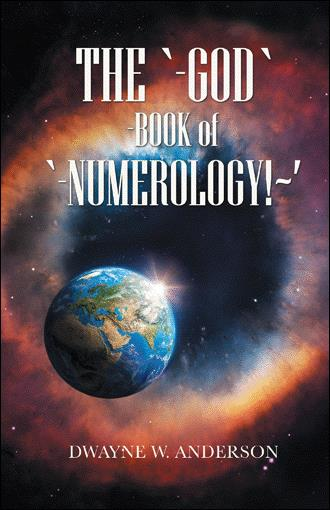# DEATHS during COVID-19 TIME!~'

Chadwick Boseman 43 was BORN in 76!~’ Birthday 11/29 / Deathday 8/28 = 11 + 29 + 8 + 28 = 76!~’ Death/Day # NUMBER = 8 + 28 + 20 + 20 = 76!~’ 7 x 6 = 42!~’ 7 + 6 = 13 = RECIPROCAL = 31!~’ 13 + 31 = 44!~’ 42 + 44 = 86 / Divided by 2 = 43 = “AGE of DEATH” for the “BLACK PANTHER”!~’

LOU BROCK 81 = RECIPROCAL = 18 = DAY of BIRTH!~’ From BIRTH to DEATH = 81 DAYS!~’ DIED at the AGE of 81!~ Birthday = 6/18 = 6(1 + 8) = 69 = RECIPROCAL = 96 = DAY of DEATH = SEPTEMBER 6th!~’ Fragmented Birthday # = 37!~’ Fragmented Deathday # = 19!~’ 37 – 19 = 18 = RECIPROCAL = 81

78Basketball Coach JOHN THOMPSON died at the AGE of 78!~’ Death/Day # = 8 + 30 + 20 + 20 = 78!~’ Singer HELEN REDDY died at the AGE of 78!~’ Death/Day # = 9 + 29 + 20 + 20 = 78!~’ American Singer/Songwriter MAC DAVIS died at the AGE of 78!~’ Death/Day # = 9 + 29 + 20 + 20 = 78!~’

Attorney MARTIN D. GINSBURG died at the AGE of 78 = RECIPROCAL = 87 = AGE of DEATH of WIFE SUPREME COURT JUSTICE RUTH BADER GINSBURG!~’ 8 x 7 or 7 x 8 = 56 = They were MARRIED for 56 YEARS!~’ MARTIN’S Birthday # = 67!~’ RUTH’S Deathday # = 67!~’ MARTIN GINSBURG died on 6(-2-)7!~’

SUPREME COURT JUSTICE RUTH BADER GINSBURG’s day OF death = 9/18 = 9 x 18 = 162!~’ ATTORNEY MARTIN D. GINSBURG’s day OF death = 6/27 = 6 x 27 = 162!~’ 162 = 62 + 1 = 63!~’ MARTIN’S DEATH/DAY # = 6 + 27 + 20 + 10 = 63!~’ 9 + 18 + 6 + 27 = 60 = MARTIN’S BIRTHDAY = 6/10 = 6 x 10 = 60

ATTORNEY MARTIN D. GINSBURG BIRTH/YEAR = 19/32 = 19 + 32 = 51 = RECIPROCAL = 15 = WIFE RUTH BADER GINSBURG’S DAY of BIRTH = 15!~’ RUTH BADER GINSBURG’s BIRTHDAY = 3/15 = 3 x 15 = 45 = RECIPROCAL = 54 = WAS MARRIED in 54!~’ MARTIN’S DEATHDAY 6/27 = 6 + 27 = 33 = RUTH’S BIRTH/YEAR!

Baseball Pitcher BOB GIBSON birth/year = 19/35 = 1 + 9 / 3 – 5 = 10/2 = his DAY of DEATH!~’ BOB GIBSON’S BIRTHDAY = 11/9 = 11 + 9 = 20!~’ HE died in 20/20!~’ DEATH/DAY = 10/2 = 10 x 2 = 20 = DIED in 20/20!~’ 11 x 9 = 99!~’ 10 x 2 = 20!~’ 99 + 20 = 11/9 = BIRTHDAY = NOVEMBER 9th!~’

Two GREATS LOU BROCK was born in the MONTH of 6 and DIED in the MONTH of 9!~’ 6/9 = RECIPROCAL = 9/6 = DAY of DEATH for LOU BROCK!~ BOB GIBSON was born in the MONTH of 11 and DIED in the MONTH of 10!~’ 11/10 = RECIPROCAL = 10/11 = 10 (1 + 1) = 10/2 = DAY of DEATH for BOB GIBSON!~

EDDIE VAN HALEN deathday # = 10/6/20/20 = 10 + 6 + 20 + 20 = 56 = RECIPROCAL = 65 = AGE of DEATH! BORN in 1955 = 19/55 = (6 =RECIPROCAL= 9) = 1 x 6 / 5 + 5 = 6/10 = RECIPROCAL = 10/6 = DAY of DEATH! VALERIE BERTINELLI birthday = 4/23/19/60 = 4 + 23 + 19 + 60 = 10/6 = DAY of DEATH

BOOK TITLE = “The GOD BOOK of NUMEROLOGY”!~’

AUTHOR: DWAYNE W. ANDERSON….

NEW RELEASE!!!~’